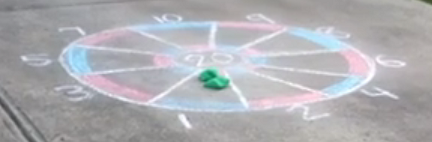Match Fishtank is now Fishtank Learning!

# Multiplication and Division of Whole Numbers

## Objective

Write expressions that represent real-world situations and evaluate them.

## Common Core Standards

### Core Standards

?

• 5.OA.A.1 — Use parentheses, brackets, or braces in numerical expressions, and evaluate expressions with these symbols.

• 5.OA.A.2 — Write simple expressions that record calculations with numbers, and interpret numerical expressions without evaluating them. For example, express the calculation "add 8 and 7, then multiply by 2" as 2 × (8 + 7). Recognize that 3 × (18932 + 921) is three times as large as 18932 + 921, without having to calculate the indicated sum or product.

?

• 3.OA.D.8

• 4.OA.A.3

## Criteria for Success

?

1. Make sense of a three-act task and persevere in solving it (MP.1).
2. Write an expression or equation to represent a real-world context, including using a letter to represent an unknown (MP.2, MP.7).
3. Evaluate an expression that represents a real-world context (MP.2, MP.7).
4. Interpret an expression that represents a real-world context without evaluating it (MP.2).

## Tips for Teachers

?

For the Problem Set, let students work on each problem on the Problem Set independently and circulate to see whether students are solving correctly. If not, come back together to discuss how/what to draw on a tape diagram and how to represent that tape diagram with an expression, then allow them to try again on their own.

#### Remote Learning Guidance

This lesson does not have any identified priority Anchor Tasks, but students should complete the Problem Set independently rather than skipping the lesson entirely. Find more guidance on adapting our math curriculum for remote learning here.

#### Fishtank Plus

• Problem Set
• Student Handout Editor
• Vocabulary Package

?

### Problem 1

Act 1: Watch following video: The Beanbag Dartboard Round 1 Act One.

How do you score points in this game? How many points did the girl get?

#### References

Illustrative Mathematics Video Game Scores

Video Game Scores, accessed on March 20, 2018, 3:23 p.m., is licensed by Illustrative Mathematics under either the CC BY 4.0 or CC BY-NC-SA 4.0. For further information, contact Illustrative Mathematics.

Modified by Fishtank Learning, Inc.

### Problem 2

Act 2: Use the following information to solve:The child earned 44 points in total.

Write expressions that could represent how the student earned all 44 points.

#### References

Illustrative Mathematics Video Game Scores

Video Game Scores, accessed on March 20, 2018, 3:23 p.m., is licensed by Illustrative Mathematics under either the CC BY 4.0 or CC BY-NC-SA 4.0. For further information, contact Illustrative Mathematics.

Modified by Fishtank Learning, Inc.

### Problem 3

Act 3: Reveal the answer by watching the video, The Beanbag Dartboard Round 1 Act Three.

#### References

Illustrative Mathematics Video Game Scores

Video Game Scores, accessed on March 20, 2018, 3:23 p.m., is licensed by Illustrative Mathematics under either the CC BY 4.0 or CC BY-NC-SA 4.0. For further information, contact Illustrative Mathematics.

Modified by Fishtank Learning, Inc.

### Problem 4

Act 4 (The Sequel): The girl played another round. She represented her tosses with the equation $(3 \times 5) + 2 \times (1 \times 8) + (2 \times 4) = s$, where $s$ represents the student’s final score.

1. What was the student’s final score?
2. What must the student have tossed?

#### References

Illustrative Mathematics Video Game Scores

Video Game Scores, accessed on March 20, 2018, 3:23 p.m., is licensed by Illustrative Mathematics under either the CC BY 4.0 or CC BY-NC-SA 4.0. For further information, contact Illustrative Mathematics.

Modified by Fishtank Learning, Inc.

## Problem Set & Homework

#### Discussion of Problem Set

• Look at #2. Did anyone solve without finding the total amount earned by both Natalie and Drew? How did you do it?
• Look at #3. What expression did you write? How did you use that to figure out what may have happened in Leila’s game?
• Look at #4. What expression did you write for Part (a)? Is there more than one correct answer? How did your expression change for when Mr. Lee doubled the number of boxes?

?

A jar with 64 fluid ounces of water is used to fill cups. The jar is used to fill 3 cups each with 8 fluid ounces of water and 2 cups each with 9 fluid ounces of water.

• Write an expression that represents the number of fluid ounces left in the jar after filling all of the cups.

A different jar has 42 fluid ounces of water. All of the water in the jar is used to fill cups. Write an equation to show how many cups can be filled if each cup is filled with 7 fluid ounces of water. Use p as the unknown number of cups in your question. Do not solve the equation.

#### References

PARCC Released Items Math Spring Operational 2016 Grade 3 Released ItemsQuestion #34

Modified by Fishtank Learning, Inc.

### Mastery Response

?